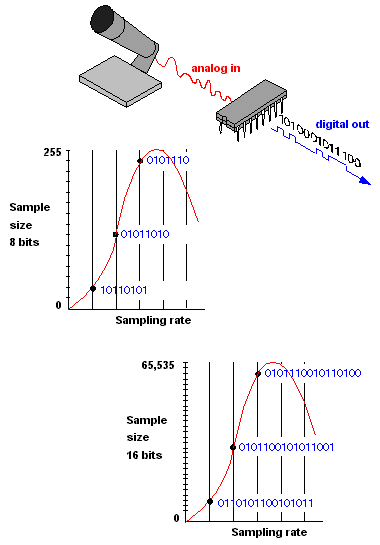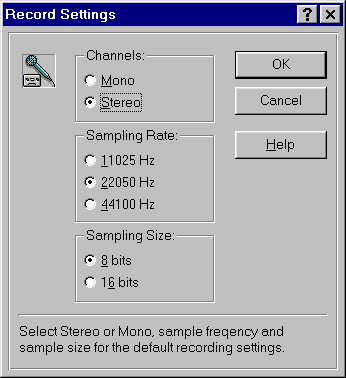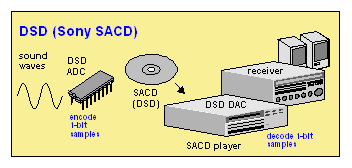# sample size

Also found in: Dictionary, Thesaurus, Medical, Financial, Acronyms, Wikipedia.
Related to sample size: sampling techniques

## sample size

[′sam·pəl ‚sīz]
(statistics)
The number of objects in the sample.

## high-resolution sampling rates

Following is a list of common digital sample sizes and sampling rates for high-quality audio. See high-resolution audio, DSD and high-resolution audio sources.

```Sample                         Stereo  Size     Sampling Rate       Transfer (Bits)  Samples Per Second      Rate

16     44,056 (44.1 kHz)**  1409 kbps

16     48,000 (48 kHz)      1536 kbps

24     88,200 (88.2 kHz)    4234 kbps

24     96,000 (96 kHz)      4608 kbps

24    176,400 (176.4 kHz)   8467 kbps

24    192,000 (192 kHz)     9216 kbps

24    384,000 (384 kHz)    18432 kbps

Compressed MP3 files (for comparison)

Common MP3 file:    128 kbps

High-res MP3 file:  320 kbps

** Standard music CD (see  CD-DA)
```

## sampling

(1) In statistics, the analysis of a group by determining the characteristics of a significant percentage of its members chosen at random.

(2) Converting analog signals into digital form. Audio and other analog signals are continuous waveforms that are analyzed at various points in time and converted into digital samples. The accuracy with which the digital samples reflect their analog origins is based on "sampling rate" and "sample size." See A/D converter.

Sampling Rate - When to Measure
The sampling rate is the number of times per second that the waveform is measured, which typically ranges from 8 to 192 thousand times per second (8 kHz to 192 kHz). The greater the rate, the higher the frequency that can be captured. For a comparison of high-quality samples, see high-resolution sampling rates.

The sampling rate must be at least twice that of the analog frequency being captured. For example, the sampling rate used to create the digital data on a CD is 44.1 kHz, slightly more than double the 20kHz frequency an average person can hear. The sampling rate for digitizing voice for a toll-quality conversation is typically 8,000 times per second (8 kHz), twice the 4 kHz required for the full spectrum of the human voice. See analog and Nyquist theorem.

Sample Size - The Measurement
Also called "resolution" and "precision," the sample size is the measurement of each sample point on a numeric scale. Known as "quantizing," the sample point is turned into the closest whole number. The more granular the scale (the more increments), the more accurate the digital sample represents the original analog signal. See oversampling, quantization and PCM.

Sampling SoundThe faster the sampling rate and the larger the sample size, the more accurately sound can be digitized. An 8-bit sample breaks the sound wave into 255 increments compared with 65,535 for a 16-bit sample.

Sampling DialogThis recording dialog from an earlier Sound Blaster sound card shows typical sampling options for digitizing sound into Windows WAV files.

DSD - A High-Res Sampling TechniqueDirect Stream Digital (DSD) is a dramatic departure from PCM. Instead of turning samples into a number with a range of values, DSD samples are only 1-bit long (0 or 1), depending on whether the wave is moving up or down from the previous sample point (see DSD).
References in periodicals archive ?
Other approaches to selecting k may also consider the observed sample mean or alter the gradations by sample size.
You will have to convert it to a standard error yourself by dividing by [square root of n] for a crossover or multiplying by [square root of (1/[n.sub.1] + 1/[n.sub.2])] for a parallel-groups trial, where n, [n.sub.1] and [n.sub.2] are appropriate sample sizes in the study for which you are imputing the standard error.
On the other hand, Kieser and Wassmer (1996), and Julious and Owen (2006) suggested an alternative approach to accommodate the variability of sample variance for sample size determination.
One reason that tests of statistical significance should not stand alone is because of the influence of sample size. With differences in magnitude between groups and level of significance held constant, changes in sample size will affect statistical power.
But due to limitations in the sample size it was impossible to collect such a large number of samples and some adjustments in the formula were made as follows:
To the questions generated by the reader, one is focused on the calculation of the sample size, while the other two questions are focus in the method of analysis, and the reader suggests, it could be more robust.
Using the rubric interpreted from previous SQLS-R4 studies that a sample size of N=100 is adequate to produce an acceptable fit to data, the current study sought to determine the minimum sample size required to offer a good fit to data within an adequately powered study using Monte Carlo simulation (22).
The confidence interval of the standard deviation is also dependent on the sample size. As the sample size increases, our estimate of standard deviation becomes better.
In this manuscript we discuss sample size and power calculations for continuous outcomes.
Abstract--Collecting age-composition data is a critical aspect of stock assessment; however, there are no biological or statistical investigations that support optimization of the distribution of sample size across species.
The most serious problem of statistical significance testing is the sensitivity to sample size and the number of items.

Site: Follow: Share:
Open / Close